### 关键词

`call()` `apply()` `arguments` `callee` `Date()` `getFullYear()` `getMonth()` `getDate()` `getDay()` `getHours()` `getMinutes()` `getSeconds()` `getTime()` `Date.now()` `abs()` `sqrt()` `ceil()` `floor()` `random()` `pow()` `max()` `min()` `round()` `包装类` `Number()` `String()` `Boolean()` `charAt()` `charCodeAt()` `fromCharCode()` `concat()` `indexOf()` `lastIndexOf()` `slice()` `substring()` `substr()` `split()` `toLowerCase()` `toUpperCase()`

### 代码

• `call()` 可以用于函数或者方法，第一个参数填写的是`this`,第二个参数以及之后的是传入的实参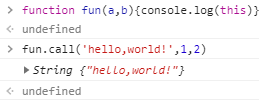• `apply()` 使用的方法和`call()`类似，但是传入实参的方法是在第二个形参位置将实参按照数组的形式传入• `agruments` 在函数或者方法中，传入的参数会以类数组对象的方式存储在这个变量内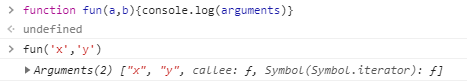• `callee` `arguments`对象的属性，用于存储当前函数或者方法的内容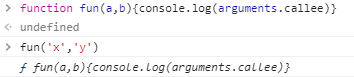• `getTime()` 获取Date对象的时间戳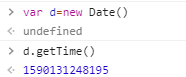• `Date.now()` 获取当前的时间戳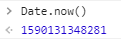• `Math.abs()` 获取绝对值
• `Math.sqrt()` 获取平方根
• `Math.pow()` 获取幂次方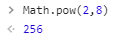• `Math.ceil()` 向上取整
• `Math.floor()` 向下取整
• `Math.random()` 取[0,1)的随机数
• `Math.round()` 取整，不是四舍五入
• `Math.max()` 多个值对比，取最大值
• `Math.min()` 多个值对比，取最小值
• `charAt()` 获取字符串指定位置的字符，和str的效果一样
• `charCodeAt()` 获取字符串指定位置的字符的Unicode编码
• `fromCharCode()` 根据Unicode编码，获取字符串字符，该方法是`String`的方法，
使用例子:`String.fromCharCode(28354) //滂`
• `concat()` 连接字符串，功能和`+`一样
• `indexOf()` 检索指定字符串第一次出现的位置，第二个参数可选，代表从什么位置开始检索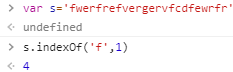• `lastIndexOf()` 功能与`indexOf()`类似，但是这个是从后面开始检索的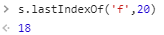• `slice` 和数组中`slice()`的方法类型，这个是字符串的方法，取值也可以为负数
• `substring()``slice()`的方法类似，但是取值不能为负
• `substr()` 也算截取字符串的作用，但是使用方法不同，第一个参数是开始截取的位置，第二个参数是截取的长度。文档中强调这方法没有在ECMAScript规范中，慎用，但是常规的浏览器都支持这一方法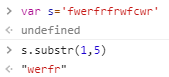• `split()` 将字符串拆分为数组，这个方法与数组中的`join()`方法相反，如果使用空值拆分，则将每一个字符串拆分成数组的每一个元素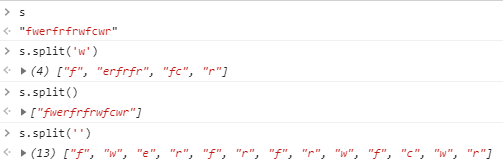• `toLowerCase()` 转换为小写
• `toUpperCase()` 转换为大写